# Geomtery - Online Test

Q1. A triangle with one angle greater than 90 degrees.
Explaination / Solution:
No Explaination.

Q2. What is the volume of this rectangular prism?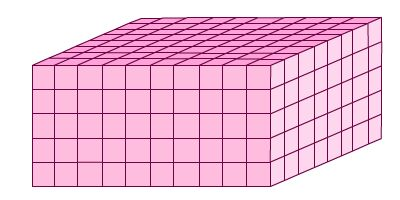Explaination / Solution:
No Explaination.

Q3. What is the missing measurement for the line segment?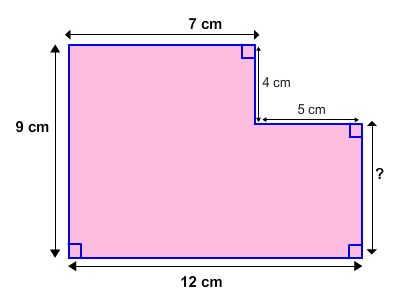Explaination / Solution:
No Explaination.

Q4. What part of the area is shaded?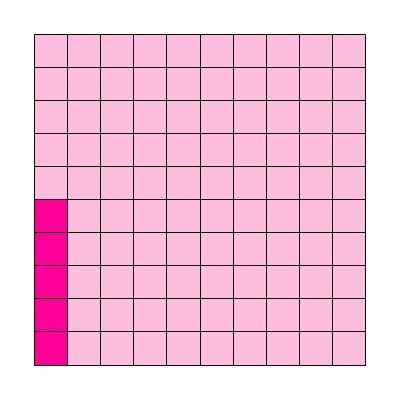Explaination / Solution:
No Explaination.

Q5. Which ordered pair represents point R?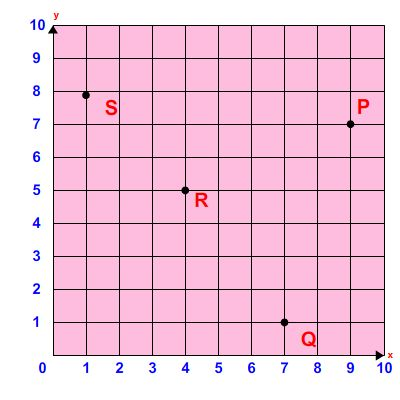Explaination / Solution:
No Explaination.

Q6. What is the sum of the interior angles of rectangle below?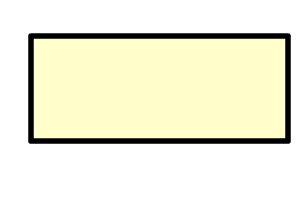Explaination / Solution:
No Explaination.

Q7. which expresson shows the area of the rectangle?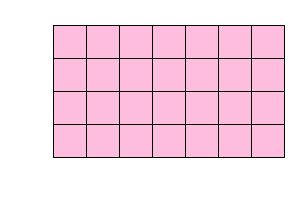Explaination / Solution:
No Explaination.

Q8. Which ordered pair represents point P?Explaination / Solution:
No Explaination.

Q9. Which ordered pair represents point R?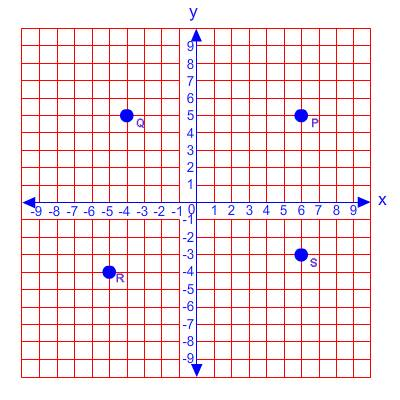Explaination / Solution:
No Explaination.

Q10. What is the best description of a point?
Explaination / Solution:
No Explaination.